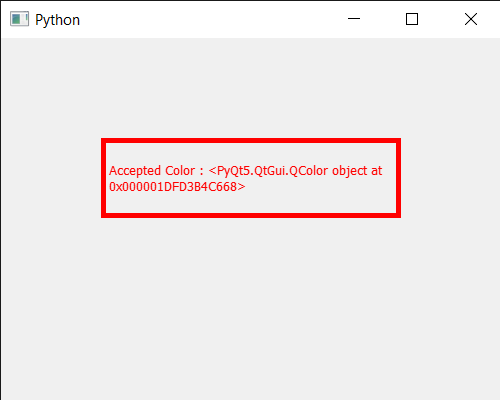# PyQt5 QColorDialog – Making color done

• Last Updated : 24 Jun, 2020

In this article we will see how we can done the color in the QColorDialog widget. This closes the dialog and sets its result code to r. The finished signal will be emitted, if r is `QDialog::Accepted` or `QDialog::Rejected`, the accepted or the rejected signals will also be emitted, respectively.

In order to do this we use `done` method with the QColorDialog object

Syntax : dialog.done(1)

Argument : It takes integer as argument if 0 is passed it will reject the color if 1 is passed it will accept the color

Return : It returns None

Below is the implementation

 `# importing libraries``from` `PyQt5.QtWidgets ``import` `*` `from` `PyQt5 ``import` `QtCore, QtGui``from` `PyQt5.QtGui ``import` `*` `from` `PyQt5.QtCore ``import` `*` `import` `sys`` ` ` ` `class` `Window(QMainWindow):`` ` `    ``def` `__init__(``self``):``        ``super``().__init__()`` ` `        ``# setting title``        ``self``.setWindowTitle(``"Python "``)`` ` `        ``# setting geometry``        ``self``.setGeometry(``100``, ``100``, ``500``, ``400``)`` ` `        ``# calling method``        ``self``.UiComponents()`` ` `        ``# showing all the widgets``        ``self``.show()`` ` ` ` `    ``# method for components``    ``def` `UiComponents(``self``):`` ` `        ``# creating a QColorDialog object``        ``dialog ``=` `QColorDialog(``self``)`` ` `        ``# setting current color to the dialog``        ``dialog.setCurrentColor(Qt.red)`` ` `        ``# making color done``        ``dialog.done(``1``)`` ` `        ``# executing the dialog``        ``# dialog.exec_()`` ` ` ` `        ``# creating label``        ``label ``=` `QLabel(``"GeeksforGeeks"``, ``self``)`` ` `        ``# setting geometry to the label``        ``label.setGeometry(``100``, ``100``, ``300``, ``80``)`` ` `        ``# making label multi line``        ``label.setWordWrap(``True``)`` ` `        ``# setting stylesheet of the label``        ``label.setStyleSheet(``"QLabel"``                            ``"{"``                            ``"border : 5px solid black;"``                            ``"}"``)`` ` `        ``# getting the selected color``        ``color ``=` `dialog.selectedColor()`` ` `        ``# setting graphic effect to the label``        ``graphic ``=` `QGraphicsColorizeEffect(``self``)`` ` `        ``# setting color to the graphic``        ``graphic.setColor(color)`` ` `        ``# setting graphic to the label``        ``label.setGraphicsEffect(graphic)`` ` `        ``# setting text to the label``        ``label.setText(``"Accepted Color : "` `+` `str``(color))`` ` ` ` ` ` `# create pyqt5 app``App ``=` `QApplication(sys.argv)`` ` `# create the instance of our Window``window ``=` `Window()`` ` `# start the app``sys.exit(App.``exec``())`

Output :In this implementation we didn’t execute the color dialog because we set the current color and accept the color programmatically will make it selected color instead of white which is default by calling the done method

My Personal Notes arrow_drop_up